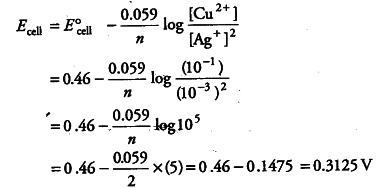# Calculate the emf of the following cell at 25°C

Calculate the emf of the following cell at 25°C
Ag(s)| \$Ag^{+}\$(\$10^{-3}\$M)|| \$Cu^{2+}\$ (\$10^{-1}\$M) Cu(s)
Given, \$ E°_{cell}\$ =+0.46 V and log \$10^{n}\$M = n.

Ag(s)| Ag^{+}(\$10^{-3}\$M)|| Cu^{2+} (\$10^{-1}\$M) Cu(s)

E°_{cell} = +0.46 V and log 10^{n} = n, T = 25°C